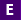# Constructing Linear Functions from TablesLogin will be required December 31, 2021 to access all the CPALMS original student tutorials. Florida public school students will continue to have access to this and all other tutorials at www.floridastudents.org or their CPALMS Class Sites. Florida public school teachers will continue to be able to access them here and on www.floridastudents.org For all other teachers, parents, and students, you can access them with a subscription on www.cpalms.com

### Attachments

Accessible Version Accessible version of the tutorial content in pdf format

### General Information

Subject(s): Mathematics, Mathematics (B.E.S.T. - Effective starting 2022-2023)
Intended Audience: Educators, Students
Keywords: Linear Functions, Functions from Tables, y=mx+b, Linear Equations, slope, y-intercept, slope intercept form, rate of change, initial value, constructing functions, functions, constructing a function, interactive, , mathematics, tutorials, elearning, e-learning
Instructional Component Type(s):

## Aligned Standards

This vetted resource aligns to concepts or skills in these benchmarks.

## Suggested Tutorials

Constructing Functions From Two Points:

Learn to construct a function to model a linear relationship between two quantities and determine the slope and y-intercept given two points that represent the function with this interactive tutorial.

Scatterplots Part 5: Interpreting the Equation of the Trend Line :

Explore how to interpret the slope and y-intercept of a linear trend line when bivariate data is graphed on a scatterplot in this interactive tutorial.

This is part 5 in 6-part series. Click below to open the other tutorials in the series.

Scatterplots Part 4: Equation of the Trend Line:

Learn how to write the equation of a linear trend line when fitted to bivariate data in a scatterplot in this interactive tutorial.

This is part 4 in 6-part series. Click below to open the other tutorials in the series.

## Related Resources

Other vetted resources related to this resource.